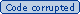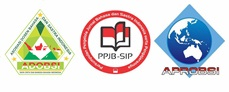### PENGARUH BAHAN AJAR BERBASIS KOMPUTER TERHADAP HASIL BELAJAR SISWA PADA MATA PELAJARAN BAHASA INDONESIA DI SMA 1 JEKULO KUDUS

bambang winanto raharjo
ENNY DWI LESTARININGSIH

#### Abstract

The research problem is how the significance level of the influence of computer-based teaching materials on students’learning outcomes in Indonesian subjects in SMA 1 Jekulo. This study used an experimental research with two variables, namely independent variables (computer-based teaching materials) and dependent variable (student learning outcomes). The research design used Control Group Pre-Test-Post-Test. To know the level of significance of the influence of computer-based teaching materials on the students’ learning achievement used t-Test: Paired Two Sample for Means. The result of pretest hypothesis test on homogeneity test obtained Fhitung = 1.10 and Ftable = 1.72 so Fcount <Ftable. This means that the initial value is homogeneously distributed. Thus, based on the initial scores of students' abilities the experimental and control groups are almost equal. In the pretest significance shown that the experimental group and the control group was not significant with tcount = 1.839 and ttable = 2.028 so that tcal < = 0.01. This means thitung was a = 0.05, and ttable = 2.39 for a ttabel.After held post-test as the end of activity obtained the correlation number was 0.618. If consulted with the table, then the number showed a high relationship. The result of t test was calculated = 12.652, whereas ttable = 2.028 for >  = 0.01. Thus, it can be concluded that the use of computer-based teaching materials has a significant effect on students’ learning outcomes.a = 0,05 or a ttabel either real level.

Keywords: teaching materials, computers, learning outcomes

#### Full Text:

PDF (Bahasa Indonesia)

DOI: https://doi.org/10.24176/kredo.v1i2.1949

#### Article MetricsAbstract views : 192|PDF (Bahasa Indonesia) views : 326### Refbacks

• There are currently no refbacks.

Copyright (c) 2018 KREDO : Jurnal Ilmiah Bahasa dan SastraView My Stats